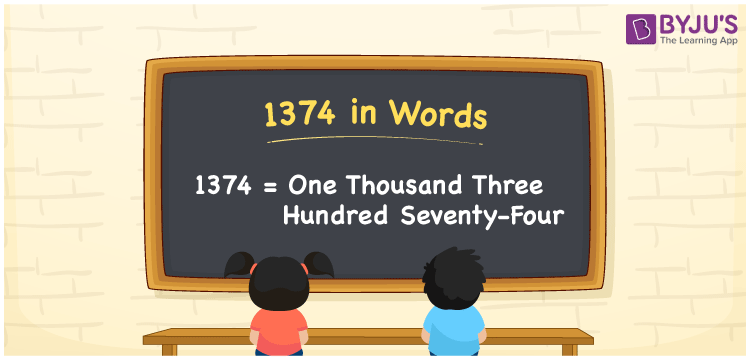# 1374 in Words

1374 in words is one thousand three hundred seventy-four. For example, if you invested Rs. 1374 on purchasing fantasy books, you can write it as “I invested Rs. One thousand three hundred seventy-four on purchasing fantasy books”. Also, the number 1374 is a cardinal number. Now, let us discuss the procedure of writing the number 1374 in words using the place value system.

 1374 in Words: One thousand three hundred seventy-four. One thousand three hundred seventy-four in Numerical Form: 1374.

## 1374 in English Words## How to Write 1374 in Words?

For the number 1374, the place value table is depicted below up to 4 digits.

 Thousands Hundreds Tens Ones 1 3 7 4

The expanded form of 1374 is as follows:

= 1 × Thousand + 3 × Hundred + 7 × Ten + 4 × One

= 1 × 1000 + 3 × 100 + 7 × 10 + 4 × 1

= 1000 + 300 + 70 + 4

= 1374

= One thousand three hundred seventy-four

Hence, 1374 in words is one thousand three hundred seventy-four.

1374 in words – One thousand three hundred seventy-four

Is 1374 an odd number? – No

Is 1374 an even number? – Yes

Is 1374 a perfect square number? – No

Is 1374 a perfect cube number? – No

Is 1374 a prime number? – No

Is 1374 a composite number? – Yes

## Frequently Asked Questions on 1374 in Words

Q1

### Write 1374 in words.

1374 in words is one thousand three hundred seventy-four.

Q2

### Simplify 1300 + 74, and express it in words.

Simplifying 1300 + 74, we get 1374. Hence, 1374 in words is one thousand three hundred seventy-four.

Q3

### Is 1374 an even number?

Yes, 1374 is an even number.